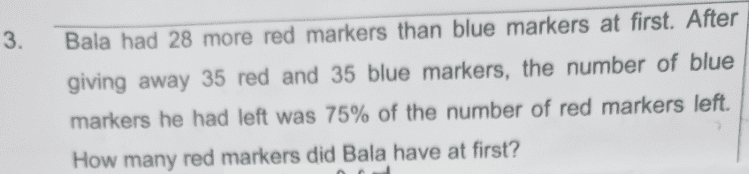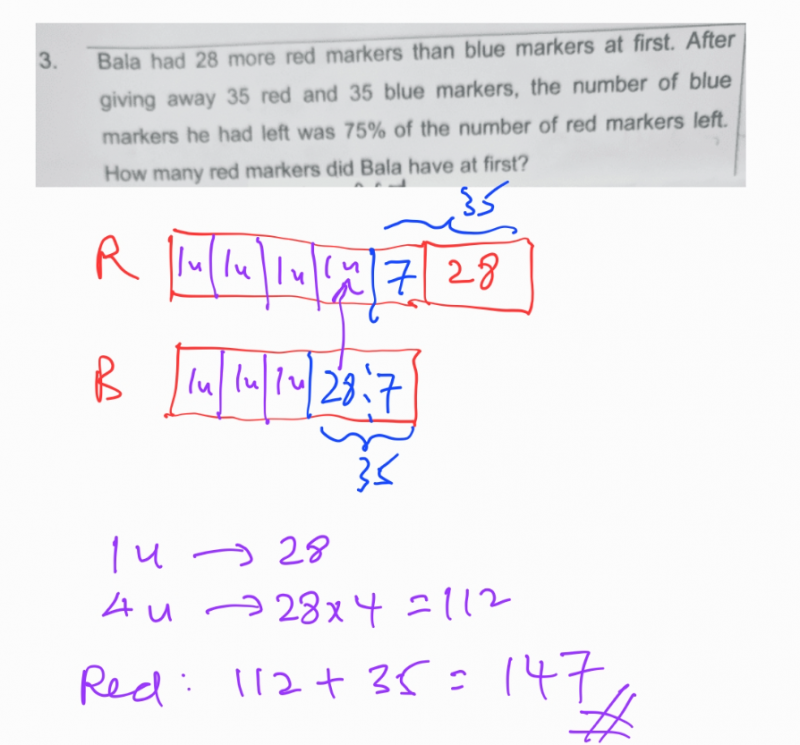# QuestionThank you0 Replies 1 Like

Let R be the original number of Red markers.
Let B be the original number of Blue markets.

R – B = 28
=> R = 28 + B

Now, 75/100(R – 35) = B – 35
R – 35 = (100/75) B – (100/75)35
=> R = (100/75) B – (100/75)35 + 35

Substituting (1) into (2):
28 + B = (4/3)B – (4/3)35 + 35
=> B/3 = 28 + (4/3)35 – 35
=> B/3 = (4/3)35 – 7
=> B = (4)35 – 21 = 119

So R = 28 + 119 = 147 #

0 Replies 1 Like